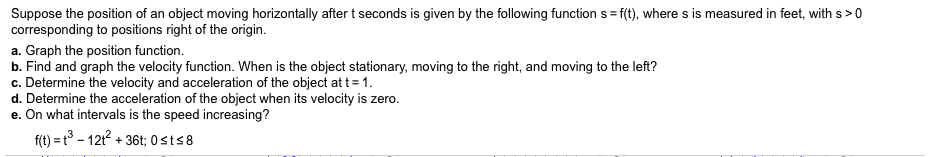# Suppose the position of an object moving horizontally after t seconds is given by the following function s = f(t), where s is measured in feet, with s>0corresponding to positions right of the origin.%3Da. Graph the position function.b. Find and graph the velocity function. When is the object stationary, moving to the right, and moving to the left?c. Determine the velocity and acceleration of the object at t= 1.d. Determine the acceleration of the object when its velocity is zero.e. On what intervals is the speed increasing?f(t) = t° - 12t2 + 36t; 0sts8

Question
8 viewshelp_outlineImage TranscriptioncloseSuppose the position of an object moving horizontally after t seconds is given by the following function s = f(t), where s is measured in feet, with s>0 corresponding to positions right of the origin. %3D a. Graph the position function. b. Find and graph the velocity function. When is the object stationary, moving to the right, and moving to the left? c. Determine the velocity and acceleration of the object at t= 1. d. Determine the acceleration of the object when its velocity is zero. e. On what intervals is the speed increasing? f(t) = t° - 12t2 + 36t; 0sts8 fullscreen
check_circle

Step 1

Note: Since we only answer up to 3 sub-parts, we’ll answer the first 3. Please resubmit the question and specify the other subparts (up to 3) you’d like answered

Step 2

Suppose the position of an object moving horizontally after t seconds is given by the following function s=f(t) , where s is measured in feet, with s>0 corresponding to positions right of the origin.

1. Graph the position function
2. Find and graph the velocity function. When is the object stationary, moving to  the right and moving to the left
3. Determine the velocity and acceleration of the object at t=1
4. Determine the acceleration of the object when its velocity is zero.
5. On what interval is the speed increasing

F(t)= t3-12t2 +36t;  0 <=t <=8

Step 3

Graph s(t) = t3-12t2 +3...

### Want to see the full answer?

See Solution

#### Want to see this answer and more?

Solutions are written by subject experts who are available 24/7. Questions are typically answered within 1 hour.*

See Solution
*Response times may vary by subject and question.
Tagged in

### Other